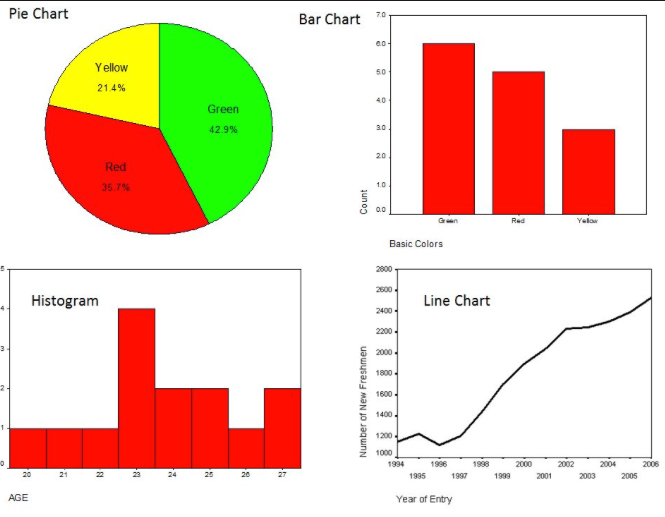# Module 4: What is Statistics?

This week the class is looking at Statistics and the differences between Descriptive and  Inferential Statistics as well as Predictive Analytics. In the various readings defining Descriptive Statistics (DS) is straightforward and easy. In DS we are simply describing the data. This description is usually done with a combination of textual description as well as the use of tables and graphical tools such as the Pie Chart, Bar Chart, Line Chart and Histogram in the figure below. We might look for something such as the Mean, Mode or Median in the data set. These are differing descriptions of Central Tendency, each is found by a different rule or formula and some types of data are described better using one of the three rather than any other. For example if we looked at the mode for the Pie Chart we would want the value that is the most frequent and that is Green, it is also the mode for the Bar Chart. However, is it the mean or the median?The mean of a data set is found by finding the sum of the data values and then dividing by the total number of data values. But this doesn’t make sense with the Bar Chart and the Pie Chart, colors are not values that can be summed up. The mean could make sense with the Histogram. Here we have a range of ages from 20-27, the mode is age 23 with 4 occurrences but is that the mean age, or more commonly the average age of this group of values? To find the mean we sum the values and divide by the total number of values so:

20+21+22+23+23+23+23+24+24+25+25+26+27+27=313 and 313/14 = 22.2571429 but looking at the histogram, does the mean or average age of 22.26 make sense with 11 of 14 23 years or more? Not really so perhaps the median age will paint a truer picture of this data.

The median is essentially the center point of the data values so in the Histogram the data set contains the 14 values 20-21-22-23-23-23-23-24-24-25-25-26-27-27. The median is the center value, since it is an even number set the two center values are added together and divided by 2: (23+24)/2=23.5. To me this poses a bit of a dilemma (side note: I just learned that dilemna is not a word…), the ages of this group clearly skew to 23 and above so to call the median age 23.5 seems a bit off, in this case since the ages are reported as whole numbers, I might want to round up to 24…

The three “M”s don’t really mean much in the Line Chart. This is a Time Line marking the increase in numbers of new freshmen per year. Using the time line visually describes this data fairly well. However, the values for new freshman could be added together and divided by the number of years to find an average number of new freshman per year. That number could then be compared to the actual number from each year and the difference above or below the average could reveal interesting information. Measuring the Spread of the values in a data set can also offer useful information: range, quartiles, absolute deviation, variance and standard deviation are various values that can be calculated.

This second graphic depicting support and lack of support for presidential candidate, Rick Perry, in 2012 and 2116 looks like an example of Inferential Statistics. Polls in general are inferential in nature. A sample of a population is polled and the answers are tabulated and analyzed in order to estimate what percentage is for or against something. Rick Perry gained startling popularity in mid-2011 but that quickly declined. In the years leading up to the 2016 primary, he never seemed to come close to the popularity he had in 2011.The third figure looks like it could be an example of Predictive Analytics (PA). PA is a more of a set of processes that takes known data collected through data mining and text analysis using various tools to create a predictive model. For some reason that we don’t have the data to understand, the chart below is forecasting a future downturn in the scores of both teachers and students…so sad…PA is very useful in generating models, such as hurricane forecasts and stock prices but there are always outliers or Black Swans as Nassim Nicholas Taleb calls them in his book. The general premise is that Black Swans are real and important, and unpredictable; it is our tendency to dismiss them that is troublesome.